Syntactic Disambiguation with
Weighted Extended Subcategorization Frames

A. F. Gelbukh

Natural Language Laboratory, Center for Computing Research (CIC), National Polytechnic Institute (IPN),
Av. Juan de Dios Bátiz, esq. Mendizabal, Zacatenco, C.P. 07738, Mexico D.F., Mexico.
gelbukh(?)pollux.cic.ipn.mx

A method of syntactic disambiguation driven by a subcategorization frames dictionary is proposed. The method relies on two probabilities for each subcategorization frame: to appear in the text, and to appear in a wrong variant built by the parser. The procedure of learning these probabilities from unprepared text corpus is described. It is based on iterative reestimation of the probabilities of the syntactic structure variants of the phrases in the corpus at one pass, and of the statistical weights of the subcategorization frames at the other pass. The dictionary automatically compiled with this method is also useful in text generation.

Key words: natural language processing, syntactic analysis, disambiguation, subcategorization frame, text generation.

Introduction

The article deals with the type of syntactic ambiguity observed in the sentence “They moved their office from the town to the capital”, seeFigure 1. Syntactic ambiguity is one of the most difficult problems of text processing. One of the most common sources of such ambiguity is attachment of prepositional phrases (or, in languages with grammatical cases, of clauses in oblique cases).

We discuss a kind of a combinatorial dictionary useful both for resolution of ambiguity related to the use of prepositions (or grammatical cases) in text analysis and for text generation. We propose an iterative procedure that in alternating steps (1) automatically acquires such a dictionary from a large text corpus and (2) resolves the syntactic ambiguity in this corpus. The dictionary can be used later for disambiguation of other texts or for text generation.

On the one hand, there was a significant interest in the last years to the problem of prepositional phrase attachment (ChurchandPatil 1994), mainly on English material, involving both rule-based approach (BrillandResnik 1994) and statistical lexical approaches (Collins and Brooks 1995; Merlo et al. 1997). On the other hand, various iterative and reestimation methods were used to calculate the probabilities for hidden Markov models, such as Baum-Welch reestimation method (Baum 1972), or to learn the grammar information from a corpus (Pereira and Schabes1992). In our work, we apply the latter techniques to the former task. While the mentioned works are based mainly on “tagging” approach to parsing, in our paper we investigate the general problem of syntactic disambiguation, i.e., of choosing one of the possible syntactic trees for the whole phrase. We also introduce the idea of taking into account the probabilities of the typical errors made by the parser, in addition to the probabilities of natural language constructions.

To keep the size of the article reasonable, the following issues are not discussed: (1) passive transformations, impersonal and reflexive constructions, (2) the handling of morphological ambiguity, (3) English attributive noun chains.

# 1.Weighted subcategorization frames

Our method relies on a dictionary that for each word lists the possible combinations of its complements. Table 1 illustrates an abridged example of a dictionary entry. (This is not a real output of the program: though we worked with Spanish and Russian texts, we are using artificial English examples in this article. The numbers are only illustrative. The last line illustrates a wrong pattern.) The symbol ‘Æ’ denotes the direct object, the symbol ‘—’denotes absence of arguments. In this example, we do not consider the subject. No semantic information is considered in this example, though in a real dictionary, some semantic features can be used together with the prepositions.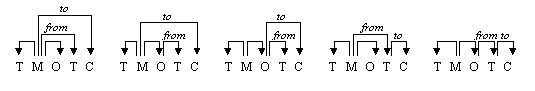Figure 1. Five variants of the syntactic structure: They (T) moved (M) their office (O) from the town (T) to the (C) capital.

With each combination, the number of its occurrences in the text corpus, denoted by n+, is included. More precisely, this is not exactly the number of occurrences; instead, as it is explained below, this is the accumulated probability to be true of all variants of the syntactic structure in which this combination occurs (for unambiguous phrases, this is the number of occurrences). More interesting is the second field, n. This is roughly the number of occurrences of the given combination in the incorrect variants of the structure built by a specific parser; more precisely, this is the accumulated probability to be false by all variants in which this combination occurs.

For example, if the parser builds five variants for the phrase shown on Figure 1, their probabilities are equal, and the corpus contains only this one phrase, then the pattern {town to} is assigned the values p+ = 2/5 and p = 8/5. Indeed, it occurs in two variants whose probabilities to be true are 1/5 and to be false 4/5. However, if it is known that the first variant is correct, then for the same pattern p+ = 0 and p = 2.

# 2.       Disambiguation with weighted subcategorization frames

Let us suppose that the parser builds some variants of the syntactic structure for each phrase, and the correct variant is always present in its output (possibly with some additional incorrect variants). By disambiguation, we mean assigning to the variants the weights according to the probability for the given variant to represent the correct structure of the phrase.

For our algorithm, each variant is represented as a set of features observed in this variant. Such features can be of different nature (say, word combinations or grammar rules). In this article we consider a combination of a word with all prepositions it governs to be a single feature. Thus, each line of Table 1 represents one possible feature. Figure 2 illustrates how a syntactic tree (one variant of the syntactic structure) is broken down into a set of features. Our formulae, however, do not depend on the specific nature of the features used.

Let p+ denote the probabilities for a given feature to occur in a true variant, and p in a false one (as we will see later, these values correspond to n+ and n in the Table 1). In this section, we will estimate the probability of the hypothesis Hi that the variant number i is the true one, given the values p+ and p. Let us call this probability the weight wi of the variant Vi. We suppose that the repertoire of all possible features is large enough so that for each specific feature, the probability not to be found in a specific variant is near to 1. Under this assumption, a simple reasoning based on the Bayes theorem gives the following (strictly speaking, approximate) formula: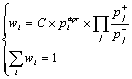(1)

where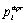is the a priori probability of this variant; C is the normalizing constant. The product is calculated by all features j found in the variant number i; the summation is done by all variants i of the same phrase. The constant C depends on the phrase, but not on the variant i. Note that the problem of zero division never occurs due to the constant l that will be introduced later in the section 3.

The corresponding algorithm for assigning the weights to the hypotheses of the syntactic structure of one phrase is as follows:

Table 1. An entry of the dictionary used for disambiguation: the word move.

 n+ n– Combination Example 8892 3782 — Jill moved impatiently. 3478 921 to John  moved to the new apartment. 372 123 Æ + from + to The firm moved its office from the town  to the capital. 135 342 Æ + out of She moved the table out of the room. 83 58 Æ + into We moved the device into the house. 76 782 to + for The family moved to the South for sake of the child. 34 89 Æ + from + through He moved the table from the room through the door. 30 219 to + at Jack moved to the new apartment at five o’clock. 25 38 to + through She moved to the South through the forest. 9 13 towards The group moved towards the mountain. 1 463 of She moved the table of John’s friend.

1.          The corpus is parsed, i.e., all variants Vi permitted by the grammar used by the parser are built for each phrase.

2.          Any available knowledge is applied to estimate a priori the quality (probability)of each variant. This procedure can take into account, say, the length of the links (the shorter links are generally scored better), semantic coherence of the structure (Gelbukh 1997), weights of the grammar rules used in it (Allen 1995, section 7.6), etc. If no information of such kind is available,are considered to be equal for all variants. For all variants, wi is set to.

3.          Each syntactic tree is broken down into features, see Figure 2. For each feature, the weights p+ and p are retrieved from the dictionary and the weight wi of the variant Vi is multiplied by pp. If the feature is not found in the dictionary, some very little value e is used.

4.          For each phrase of the corpus, the weights wi of its variants are normalized so that S wi = 1 with summation by the variants of the structure for this one phrase only.

# 3.       Learning weighted subcategorization frames from a corpus

Now let us consider the opposite task. Given a parser and a disambiguation procedure that assigns the weights wi to the variants Vi, let us calculate the probabilities p+ and p for the features found in this corpus.

If the disambiguation procedure picked up one variant as definitely true and marked all the other ones as definitely false, for each feature the probabilities p+ and p would be the normalized frequencies of its occurrence in the correct and wrong variants, correspondingly. Since in this case the weights wi would be 1 for the correct variants and 0 for the wrong ones, the corresponding numbers of occurrences (that only differ from p+ and p by a normalizing constant) would be n+ = S wi, n = S (1 – wi), with summation by all the variants that contain this feature.

However, in our case the weights wi Î [0, 1]. It can be shown, again with the Bayes theorem, that the same formulae can be used in this case. To calculate the probabilities, the total numbers  n+ and n are divided by the number of the true and false variants in the corpus, correspondingly. Fortunately, these numbers are known exactly. Let V be the number of the variants generated by the parser and S the number of sentences in the corpus, then the total number of the correct variants is S and of incorrect ones V – S. Thus, the formulae for the probabilities are the following:(2)

where the meaning of a small value l is described in the next paragraph. The summation is done by all variants Vi that contain the feature j.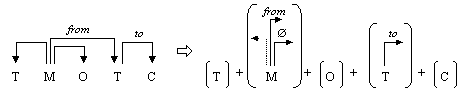Figure 2. Breaking a syntactic tree down into a set of features.

Without l (i.e., with l = 0), these formulae work in the ideal case when the corpus is so big that any possible feature occurs in it at least once. In reality, due to the great variety of the constructions in open texts, all possible words and combinations cannot occur in any corpus, even very large. The features for which n = p = 0 present a zero division problem for the formula (1), and the features for which n is too little introduce instability in the calculations. To solve these problems, introduction of a relatively small value l » S can be justified by the formula of Laplace for Bayesian estimates; for the features with high frequencies this value does not greatly affect the probability p, but it does suppress the influence of the rare features. Though Collins and Brooks (1995) especially emphasized the significance of the rare cases, we did not observe such an effect, with smoothing out the rare cases giving much better results in our experiments.

The algorithm for accumulating the probabilities p+ and p of the features is as follows:

1.          The corpus is parsed, i.e., all variants Vi permitted by the grammar used by the parser are built for each phrase, and the variants for each phrase are estimated as it was described in the previous section, i.e., are assigned the weights wi such that S wi = 1 for each phrase.

2.          For each variant i and each feature j found in it, the countersand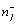are incremented by the values wi and 1 – wi, respectively, the initial values for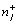andbeing zeroes.

3.          Finally,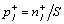andare calculated.

The combinations with the quotient p+ / p less than some threshold value e mentioned in the previous section are eliminated from the dictionary to reduce its size.

# 4.       Iterative disambiguation and learning

In the sections 2 and 3, the procedures are described working in the mutually opposite directions. For disambiguation of a large corpus, as well as for automatic acquisition of the dictionary shown in the Table 1, these two procedures are used iteratively, in alternating steps. The work starts from an empty dictionary. The disambiguation procedure (section 2) assigns equal weights to all variants (or preserves their a priori weights). At the next step, these weights are used to train the model, i.e., to determine the probabilities p+ and p (section 3). Then the process is repeated. In our experiments, the iterative process converged very quickly.

Technically, once the set of the variants and the set of features found in these variants have been built, these data structures are fixed in the computer memory, since all operations in both procedures are arithmetical and do not produce any new objects like syntactic trees or features. However, in the process of calculation or at the end, the features with too little p+ / p can be eliminated. In our experiments, after very few iterations the dictionary of features became fairly small.

# 5.       Experimental results

In our experiments, two values were measured: the similarity between the dictionary built by the program and the “true” one, and the percentage of the correctly parsed sentences. By correctly parsed sentences we mean the ones for which the variant with the weight wi highest within its phrase was the true one.

In the first set of experiments, to measure the similarity between the dictionaries a real text corpus could not be used because the “true” dictionary that the author had in mind was unknown. Thus, to check the method, we modeled the process of text generation to obtain a quasi-text corpus built with a known dictionary (Bolshakov et al. 1998). Only the statistical characteristics of the text were modeled, such as the length of the phrase and, of course, the preposition usage. With this method we were able to measure the percentage of the correctly parsed phrases as well.

In various experiments we observed all three patterns of convergence mentioned by Elworthy (1994), depending on the formulae and parameters we used, as well as on the size of the corpus. The best result – which Elworthy called the classical pattern – was achieved with l of the order of S and with a large enough corpus (of at least several thousand phrases).

To measure the nearness between the dictionary built by the algorithm and the “true” one built in the generator, we used several measures: the percentage of incorrect combinations, the coverage, and the difference of the probabilities p+ for the correct combinations. After few iterations, these values stabilized at about 95% of correct combinations and 80% of similarity of probabilities. The coverage was about 30%, probably due to insufficient size of the corpus we used.

For the measure of the correctly guessed variants, a typical sequence of the percentages was 37, 85, 89, 90, 90%, etc. (taking into account only ambiguous phrases: 16, 80, 86, 87, 87%, etc.); then the results stabilized. The first figures in both sequences were obtained with the equal weights, i.e., by picking just an arbitrary variant for each phrase. The last figures of the sentences show the accuracy reached by the algorithm.

The second set of experiments was carried out with a real Spanish 8 million word corpus. We used a very simple context-free grammar. A spot check of the results showed 78% of correctly parsed phrases; on unseen data analyzed with the dictionary built at the training stage, this figure was 69%. Note that all figures reflect not the number of correctly attached prepositions, but instead the number of correctly parsed entire sentences. With a more elaborated grammar, we expect to reach better results.

Surprisingly, in the experiments we did not observe any advantage of using some nontrivial initial values for the weights of the hypotheses or for the dictionary. The best results were obtained with equal initial weights, i.e., with initially empty dictionary. However, the a priori information can be used at each iteration, as it was described in the section 2.

# 6.Conclusions

A procedure of syntactic disambiguation driven by the dictionary of syntactic subcategorization frames with statistical weights was proposed. The dictionary can be automatically acquired from a large text corpus. The method has the following advantages:

·           No hand preparation is required to train the model. However, morphological and syntactical analyzers are required since the method disambiguates the results of such parsing.

·           The method is compatible with other methods of disambiguation, especially with methods that produce an estimation of probability for each variant (what in (1) is called apriori estimates).

·           The method is tuned to a specific parser, taking into account the balance between the correct and wrong assignments of prepositions to words.

We believe that our method has some psycholinguistic justification, i.e., that in text composition the native speakers try to avoid constructions that would be misleading with respect to the probabilities of subcategorization frames, cf.: ?They laughed at this place vs. They laughed here, ?He spoke with the director of the new plan vs. He spoke of the new plan with the director.

Acknowledgments

The work was done under partial support of REDII-CONACyT, CONACyT grant 26424-A, and SNI, Mexico.

References

Allen, James. 1995. Natural Language Understanding. The Benjamin/Cummings Publishing Company, Inc.

Collins, M., and J. Brooks. 1995. Prepositional phrase attachment through a backed-off model. Proc. of 3rd workshop on very large corpora, June 30, MIT, Cambridge, Massachusetts, USA.

Gelbukh, A.F. 1997. Using a semantic network for lexical and syntactical disambiguation. Proc. of CIC’97, Nuevas Aplicaciones e Innovaciones Tecnológicas en Computación, Simpósium internacional de computación, CIC, IPN, Mexico City.